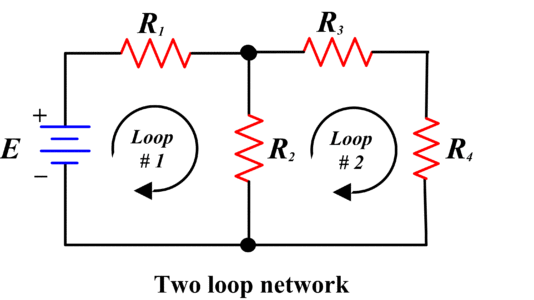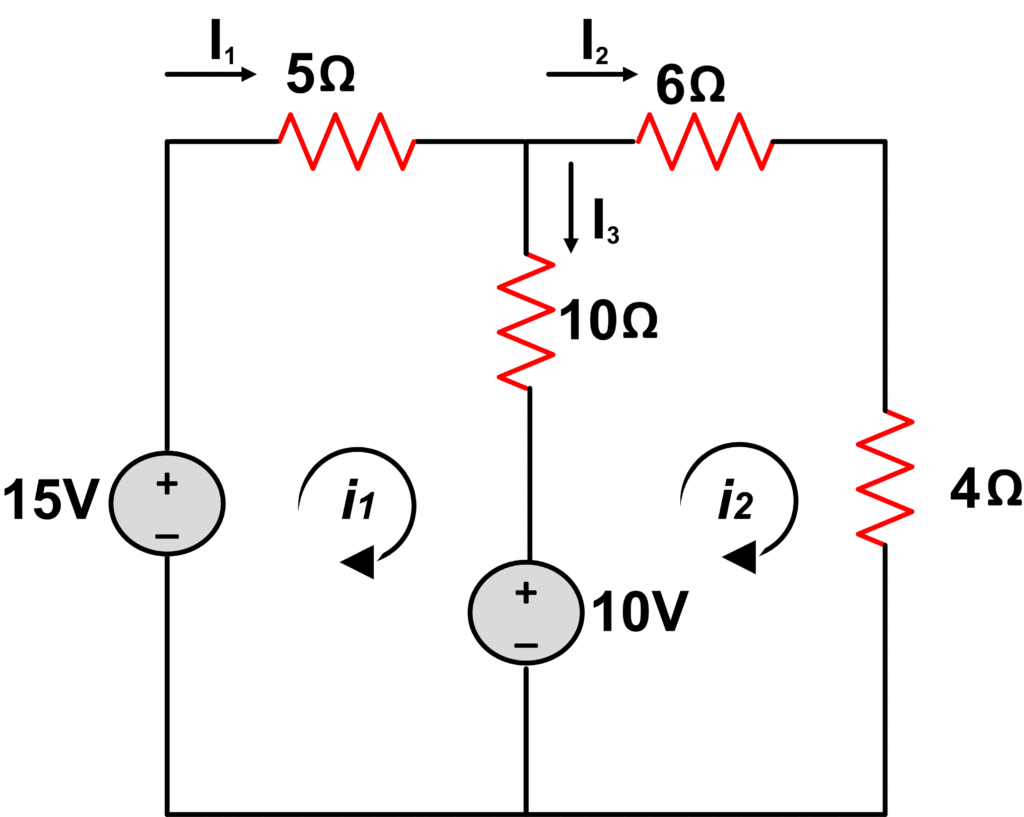# Mesh Current Analysis | Mesh Analysis

Want create site? Find Free WordPress Themes and plugins.

The mesh is a closed path which does not contain any other closed path within it.

This section shows that a set of simultaneous linear equations can be written which describes the network. This set of equations depends on a choice of loop currents used in connection with Kirchhoff’s law.

The circuit shown in the following figure has two loops:We can assign currents to represent each loop, such as Ito represent loop 1,  IB  to represent loop 2. These currents are called loop currents. Loop currents provide us with a mathematical solution for the branch currents and thus the voltage drop in a network.

A general procedure to analyze network by Mesh or loop analysis is as follows:

1. Assign a loop current for each independent closed path. The number of independent loops in a network is given by the expression.

$T-N+1$

Where T is the total number of elements in the network (both active and passive) and N the number of nodes in the network. Although the direction of loop currents is arbitrary. We shall only assign clockwise direction for the sake of uniformity.

2. Indicate the polarity markings across each passive element due to the direction of loop current in that closed path. The polarities of voltage sources are determined by their own positive and negative terminals.

3. Apply Kirchhoff’s voltage law (KVL) to each closed path. If we are applying KVL to one loop and a passive element has two or more loop currents flowing through it, the total voltage drop equals the sum of the voltage drops due to each loop current separately. If the loop currents are in the same directions, the voltage drops add. However, if the loop currents flow in the opposite directions, the voltage drops subtract from one another.

4. Solve the set of simultaneous linear equations for the loop currents.

## Example of Mesh Analysis

Find branch currents I1, I2, and I3 using mesh current method.Loop 1 Equation

$-15+5{{i}_{1}}+10+10\left( {{i}_{1}}-{{i}_{2}} \right)=0$

$-2{{i}_{2}}+3{{i}_{1}}=1~~\text{ }\cdots \text{ }~~\left( 1 \right)$

Loop 2 equation

$-10+4{{i}_{2}}+6{{i}_{2}}+10\left( {{i}_{2}}-{{i}_{1}} \right)=0$

$2{{i}_{2}}-1={{i}_{1}}~~\text{ }\cdots ~~~\left( 2 \right)$

By solving equation (1) and (2), we have

${{i}_{1}}=1A$

${{i}_{2}}=1A$

Then

${{i}_{3}}={{i}_{1}}-{{i}_{2}}$

${{i}_{3}}=0$

Note that in above circuit, the voltage source of 10V is common to both loops, so, it must be included in both loop equations.

It is important to understand that Mesh or Loop analysis can be applied to either AC resistive circuit or DC resistive circuit. In any type of network, the numbers of linear equations are dependent on the number of loop currents.

Did you find apk for android? You can find new Free Android Games and apps.